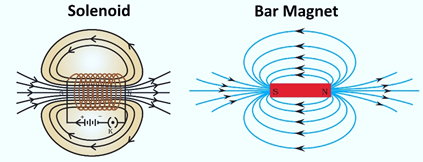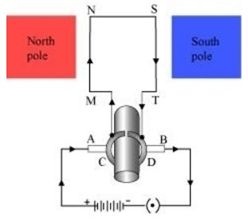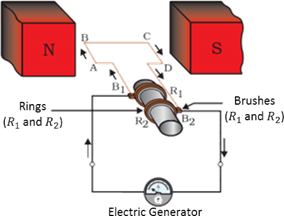# NCERT Class 10: Science-Exercise Solutions

### Chapter 13-Magnetic Effects of Electric Current-Exercise Solutions

NCERT Book Exercise-Page Number-240-241

Q.1. Which of the following correctly describes the magnetic field near a long straight wire?
(a) The field consists of straight lines perpendicular to the wire
(b) The field consists of straight lines parallel to the wire
(c) The field consists of radial lines originating from the wire
(d) The field consists of concentric circles centred on the wire
Ans. (d) The magnetic field lines, produced around a straight current-carrying conductor, are concentric circles. Their centre lies on the wire.
Q.2. The phenomenon of electromagnetic induction is
(a) the process of charging a body
(b) the process of generating magnetic field due to a current passing through a coil
(c) producing induced current in a coil due to relative motion between a magnet and the coil
(d) the process of rotating a coil of an electric motor
Ans. (c) When a coil and a magnet are moved relative to each other, a current is induced in the coil. This phenomenon is known as electromagnetic induction.
Q.3. The device used for producing electric current is called a
(a) generator (b) galvanometer
(c) ammeter (d) motor
Ans. (a) An electric generator produces electric current. It converts mechanical energy into electricity.
Q.4. The essential difference between an AC generator and a DC generator is that
(a) AC generator has an electromagnet while a DC generator has permanent magnet.
(b) DC generator will generate a higher voltage.
(c) AC generator will generate a higher voltage.
(d) AC generator has slip rings while the DC generator has a commutator.
Ans. (d) An AC generator has two rings called slip rings. A DC generator has two half rings called commutator. This is the main difference between both the types of generators.
Q.5. At the time of short circuit, the current in the circuit
(a) reduces substantially
(b) does not change
(c) increases heavily
(d) vary continuously
Ans. (c) When two naked wires of an electric circuit touch each other, the amount of current that is flowing in the circuit increases abruptly. This causes short-circuit.
Q.6. State whether the following statements are true or false.
(a) An electric motor converts mechanical energy into electrical energy.
(b) An electric generator works on the principle of electromagnetic induction.
(c) The field at the centre of a long circular coil carrying current will be parallel straight lines.
(d) A wire with a green insulation is usually the live wire of an electric supply.
Ans. (a) Flase
An electric motor converts electrical energy into mechanical energy.
(b) True
A generator is an electric device that generates electricity by rotating a coil in a magnetic field. It works on the principle of electromagnetic induction.
(c) True
A long circular coil is a long solenoid. The magnetic field lines inside the solenoid are parallel lines.
(d) False
Live wire has red insulation cover, whereas earth wire has green insulation colour in the domestic circuits.
Q.7. List two methods of producing magnetic fields.
Ans. Three sources of magnetic fields are as follows:
(a) Current-carrying conductors
(b) Permanent magnets
(c) Electromagnets
Q.8. How does a solenoid behave like a magnet? Can you determine the north and south poles of a current-carrying solenoid with the help of a bar magnet? Explain.
Ans. A solenoid is a long coil of circular loops of insulated copper wire. Magnetic field lines are produced around the solenoid when a current is allowed to flow through it. The magnetic field produced by it is similar to the magnetic field of a bar magnet. The field lines produced in a current-carrying solenoid is shown in the following figure.In the above figure, when the north pole of a bar magnet is brought near the end connected to the negative terminal of the battery, the solenoid repels the bar magnet. Since like poles repel each other, the end connected to the negative terminal of the battery behaves as the north pole of the solenoid and the other end behaves as a south pole. Hence, one end of the solenoid behaves as a north pole and the other end behaves as a south pole.
Q.9. When is the force experienced by a current-carrying conductor placed in a magnetic field largest ?
Ans. The force experienced by a current-currying conductor is the maximum when the direction of current is perpendicular to the direction of the magnetic field.
Q.10. Imagine that you are sitting in a chamber with your back to one wall. An electron beam, moving horizontally from back wall towards the front wall, is deflected by a strong magnetic field to your right side. What is the direction of magnetic field?
Ans. The direction of magnetic field is given by Fleming’s left hand rule. Magnetic field inside the chamber will be perpendicular to the direction of current (opposite to the direction of electron) and direction of deflection/force i.e., either upward or downward. The direction of current is from the front wall to the back wall because negatively charged electrons are moving from back wall to the front wall. The direction of magnetic force is to the right.
Hence, using Fleming’s left hand rule, it can be concluded that the direction of magnetic field inside the chamber is downward.
Q.11. Draw a labelled diagram of an electric motor. Explain its principle and working. What is the function of a split ring in an electric motor?
Ans. An electric motor converts electrical energy into mechanical energy. It works on the principle of the magnetic effect of current. A current-carrying coil rotates in a magnetic field. The following figure shows a simple electric motor.When a current is allowed to flow through the coil MNST by closing the switch. Current in the length MN flows from M to N and the magnetic field acts from left to right, normal to length MN. Therefore, according to Fleming’s left hand rule, a downward force acts on the length MN. Similarly, current in the length ST flows from S to T and the magnetic field acts from left to right, normal to the flow of current. Therefore, an upward force acts on the length ST. These two forces cause the coil to rotate anti-clockwise.
After half a rotation, the position of MN and ST interchange. The half-ring D comes in contact with brush A and half-ring C comes in contact with brush B. Hence, the direction of current in the coil MNST gets reversed.

The current flows through the coil in the direction TSNM. The reversal of current through the coil repeats after each half rotation. As a result, the coil rotates unidirectional. The split rings help to reverse the direction of current in the circuit. These are called the commutator.
Q.12. Name some devices in which electric motors are used?
Ans. Some devices in which electric motors are used are as follows:
(a) Water pumps
(b) Electric fans
(c) Electric mixers
(d) Washing machines
Q.13. A coil of insulated copper wire is connected to a galvanometer. What will happen if a bar magnet is (i) pushed into the coil, (ii) withdrawn from inside the coil, (iii) held stationary inside the coil ?
Ans. A current induces in a solenoid if a bar magnet is moved relative to it. This is the principle of electromagnetic induction.
(i) When a bar magnet is pushed into a coil of insulated copper wire, a current is induced momentarily in the coil. As a result, the needle of the galvanometer deflects momentarily in a particular direction.
(ii) When the bar magnet is withdrawn from inside the coil of the insulated copper wire, a current is again induced momentarily in the coil in the opposite direction. As a result., the needle of the galvanometer deflects momentarily in the opposite direction.
(iii) When a bar magnet is held stationary inside the coil, no current will be induced in the coil.
Hence, galvanometer will show no deflection.
Q.14. Two circular coils A and B are placed closed to each other. If the current in the coil A is changed, will some current be induced in the coil B? Give reason.
Ans. Two circular coils A and B are placed close to each other. When the current in coil A is changed, the magnetic fieid associated with it also changes. As a result, die magnetic field around coil B also changes. This change in magnetic field lines around coil B induces an electric current in it.
This is called electromagnetic induction.
Q.15. State the rule to determine the direction of a (i) magnetic field produced around a straight conductor-carrying current, (ii) force experienced by a current-carrying straight conductor placed in a magnetic field which is perpendicular to it, and (iii) current induced in a coil due to its rotation in a magnetic field.
Ans. (i) Maxwell’s right hand thumb rule
(ii) Fleming’s left hand rule
(iii) Fleming’s right hand rule
Q.16. Explain the underlying principle and working of an electric generator by drawing a labelled diagram. What is the function of brushes ?
Ans. An electric generator converts mechanical energy into electrical energy.
The principle of working of an electric generator is that when a loop is moved in a magnetic field, an electric current is induced in the coil. It generates electricity by rotating a coil in a magnetic field. The following figure shows a simple AC generator.ABCD → Rectangular coil
B1 and B2 → Brushes
R1 and R2 → Slip rings
X → Axle, G → Galvanometer
If axle X is rotated clockwise, then the length AB moves upwards while length CD moves downwards. Since the lengths AB and CD are moving in a magnetic field, a current will be induced in both of them due to electromagnetic induction. Length AB is moving upwards and the magnetic field acts from left to right. Hence, according to Fleming’s right hand rule, the direction of induced current will be from A to B. Similarly, the direction of induced current in the length CD will be from C to D.
The direction of current in the coil is ABCD. Hence, the galvanometer shows a deflection in a particular direction. After half a rotation, length AB starts moving down whereas length CD starts moving upward. The direction of the induced current in the coil gets reversed as CDBA. As the direction of current gets reversed after each half rotation, the produced current is called an alternating current (AC).
To get a unidirectional current, instead of two slip rings, two split rings are used.
In this arrangement, brush A always remains in contact with the length of the coil that is moving up whereas brush B always remains in contact with the length that is moving down. The split rings R1 and R2 act as a commutator.
The direction of current induced in the coil will be ABCD for the first rotation and DCBA in the second half of the rotation. Hence, a unidirectional current is produced from the generator called DC generator. The current is called DC current.

Q.17. When does an electric short circuit occur?
Ans. If the resistance of an electric circuit becomes very low, then the current flowing through the circuit becomes very high. When too many appliances are connected to a single socket or high power rating appliances are connected to the low power rated circuits. When the insulation of live and neutral wires undergo wear and tear and come into contact with each other, the current flowing in the circuit increases abruptly. Hence, a short circuit occurs.
Q.18. What is the function of an earth wire? Why is it necessary to earth metallic appliances?
Ans. The metallic body of electric appliances is connected to the earth by means of earth wire so that any leakage of electric current is transferred to the ground. This prevents any electric shock to the user. That is why earthing of the electrical appliances is necessary.